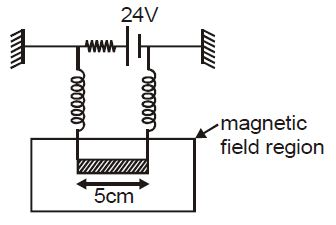# Extension in Spring due to Magnetic force!The circuit in figure consists of wires at the top and bottom and identical metal springs as the left and right sides. The wire at the bottom has a mass of $10 \text{ g}$ and is $5 \text{ cm}$ long. The wire is hanging as shown in the figure. The spring stretch $0.5 \text{ cm}$ under the weight of the wire and the circuit has a total resistance of $12 \Omega$. When the lower wire is subjected to a static magnetic field, the springs stretch an additional $0.3 \text{ cm}$. The magnetic field is?

×